6553.0 - Survey of Income and Housing, User Guide, Australia, 2017-18
Latest ISSUE Released at 11:30 AM (CANBERRA TIME) 12/07/2019
 Page tools: .mffeedback,#pjs { display :none; } Enable Javascript to Print PagesPrint AllEmail NotificationRSSSearch this Product RELIABILITY OF ESTIMATES SAMPLING VARIABILITY The estimates provided from the Survey of Income and Housing (SIH) are subject to two types of error, non-sampling and sampling error. Comparisons between estimates from surveys conducted in different periods, for example, comparison of 2017–18 SIH estimates with previous cycle estimates, are also subject to the impact of any changes made to the way the survey is conducted. For further details on changes between cycles see the 'Historical information' section of this publication. Non-sampling error Non-sampling error can occur in any collection, whether the estimates are derived from a sample or from a complete collection such as a census. Sources of non-sampling error include non-response, errors in reporting by respondents or recording of answers by interviewers and errors in coding and processing the data. Non-sampling errors are difficult to quantify in any collection. However, every effort is made to reduce non-sampling error to a minimum by careful design and testing of the questionnaire, training of interviewers and data entry staff, and extensive editing and quality control procedures at all stages of data processing. One of the main sources of non-sampling error is non-response by persons selected in the survey. Non-response occurs when people cannot or will not cooperate or cannot be contacted. Non-response can affect the reliability of results and can introduce a bias. The magnitude of any bias depends upon the level of non-response and the extent of the difference between the characteristics of those people who responded to the survey and those who did not. The following methods were adopted to reduce the level and impact of non-response: Primary Approach Letters (PALs) were posted to selected SIH households prior to enumeration document cards were provided to respondents to suggest having financial statements and similar documents handy at the time of interview to assist with accurate responses face-to-face interviews with respondents the use of interviewers who could speak languages other than English, where necessary proxy interviews conducted when consent is given, with a responsible person answering on behalf of a respondent incapable of doing so themselves follow-up of respondents if there was initially no response imputation of missing values ensuring that the weighted data is representative of the population (in terms of demographic characteristics) by aligning the estimates with population benchmarks. Sampling error The sampling error is a measure of the variability that occurs by chance because a sample, rather than the entire population, is surveyed. Since the estimates in this publication are based on information obtained from occupants of a sample of dwellings they are subject to sampling variability; that is, they may differ from the figures that would have been produced if all dwellings had been included in the survey. One measure of the likely difference is given by the standard error estimate (SE), which indicates the extent to which a sample estimate might have varied compared to the population parameter because only a sample of dwellings were included. There are about two chances in three that the sample estimate will differ by less than one SE from the population parameter that would have been obtained if all dwellings had been enumerated, and about 19 chances in 20 that the difference will be less than two SEs. Another measure of the likely difference is the relative standard error (RSE), which is obtained by expressing the SE as a percentage of the estimate. The RSE is a useful measure in that it provides an immediate indication of the percentage errors likely to have occurred due to sampling, and thus avoids the need to refer also to the size of the estimate. For estimates of population sizes, the size of the SE generally increases with the level of the estimate, so that the larger the estimate the larger the SE. However, the larger the sampling estimate the smaller the SE becomes in percentage terms. Thus, larger sample estimates will be relatively more reliable than smaller estimates. Estimates with RSEs of 25% or more are not considered reliable for most purposes. Estimates with RSEs greater than 25% but less than or equal to 50% are annotated by an asterisk (*) to indicate they are subject to high SEs and should be used with caution. Estimates with RSEs of greater than 50%, annotated by a double asterisk (**) are considered too unreliable for general use and should only be used to aggregate with other estimates to provide derived estimates with RSEs of 50% or less. RSEs for the SIH have been derived using the delete-a-group jackknife method. If needed, SEs can be calculated using the estimates and RSEs. MEASURES OF RELIABILITY Proportions and percentages Proportions and percentages, which are formed from the ratio of two estimates, are also subject to sampling errors. The size of the error depends on the accuracy of both the numerator and the denominator. For proportions where the denominator is an estimate of the number in a grouping and the numerator is the number in a subgroup of the denominator group, the formula for an approximate RSE is given by: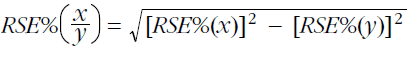Differences between estimates The difference between survey estimates is also subject to sampling variability. An approximate SE of the difference between two estimates (x–y) may be calculated by the formula: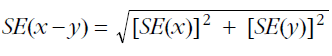This approximation can generally be used whenever the estimates come from different samples, such as two estimates from different years or two estimates for two non-intersecting subpopulations in the one year. If the estimates come from two populations, one of which is a subpopulation of the other, the standard error is likely to be lower than that derived from this approximation. Calculation of Margin of Error Another useful measure is the Margin of Error (MoE), which describes the distance from the population value that the sample estimate is likely to be within, and is specified at a given level of confidence. Confidence levels typically used are 90%, 95% and 99%. For example, at the 95% confidence level the MOE indicates that there are about 19 chances in 20 that the estimate will differ by less than the specified MOE from the population value (the figure obtained if all dwellings had been enumerated). The 95% MOE is calculated as 1.96 multiplied by the SE. A confidence interval expresses the sampling error as a range in which the population value is expected to lie at a given level of confidence. The confidence interval can easily be constructed from the MoE of the same level of confidence, by taking the estimate plus or minus the MoE of the estimate. In other terms, the 95% confidence interval is the estimate +/- MoE i.e. the range from minus 1.96 times the SE to the estimate plus 1.96 times the SE. The 95% MoE can also be calculated from the RSE by the following, where y is the value of the estimate: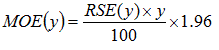Note due to rounding, the SE calculated from the RSE may be slightly different to the SE calculated from the MoE for the same estimate. The SE of estimate using MoEs is calculated by: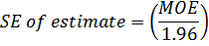In the tables in this publication, MoEs are presented for the proportion estimates (%). Proportion estimates are preceded by a hash (e.g. #10.2) if the corresponding MoE is greater than 10 percentage points. An estimate is also preceded by a hash if the MoE is large enough such that the corresponding confidence interval for this estimate would exceed the value of 0% and/or 100%; the natural limits of a proportion. The latter situation will occur if the MoE is greater than the estimate itself, or greater than 100 minus the estimate. Users should give the margin of error particular consideration when using this estimate. Significance testing When comparing estimates between surveys or between populations within a survey, it is useful to determine whether apparent differences are 'real' differences between the corresponding population characteristics or simply the result of sampling variability between the survey samples. One way to examine this is to determine whether the difference between the estimates is statistically significant. This is done by calculating the standard error of the difference between two estimates (x and y), see the 'Differences between estimates' section above, and applying that to calculate the test statistic using the formula below: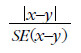If the value of this test statistic is greater than 1.96 (at the 95% confidence level) then there is good evidence of a statistically significant difference between the two population estimates with respect to that characteristic. Otherwise, it cannot be stated with confidence that there is a real difference between the population estimates. Document Selection These documents will be presented in a new window.